Courses

# Ex 1.5 NCERT Solutions - Number System Class 9 Notes | EduRev

## Class 9 : Ex 1.5 NCERT Solutions - Number System Class 9 Notes | EduRev

The document Ex 1.5 NCERT Solutions - Number System Class 9 Notes | EduRev is a part of the Class 9 Course Class 9 Mathematics by VP Classes.
All you need of Class 9 at this link: Class 9

Q.1. Classify the following numbers as rational or irrational:
(i) 2 - √5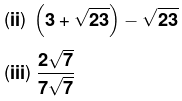(iv) 1/√2
(v) 2π
Ans.
(i) 2 –√5
Since it is a difference of a rational and irrational number,
∴ 2 –√5 is an irrational number.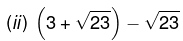We have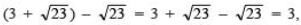which is a rational number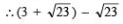is a rational number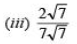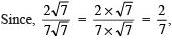which is a rational number.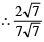is a rational number.

(iv) 1/√2
∵The quotient of rational and irrational is an irrational number.
∴  1/√2 is an irrational number.

(v)
∵ 2π = 2 x π = Product of a rational and an irrational (which is an irrational number)
∴ 2π is an irrational number.

REMEMBER
For real numbers a, b, c and d, we have: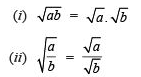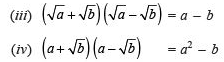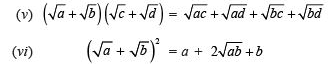Q.2. Simplify each of the following expressions:
(i) (3 + √3) (2 + √2)
(ii) (3 + √3)(3 - √3)
(iii) (√5 + √2)2
(iv) (√5 - √2) (√5 + √2)
Ans.
(i) (3+ √3)(2+√2) - 2(3 +√3) + √2 (3 +√3)
= (2 x 3 + 2√3) + (3√2 +√2 x √3)
= 6 + 2√3 + 3√2 + √6
Thus, (3 +√3)(2 +√2) = 6 + 2√3 + 3√2 +√6
(ii) (3 +√3)(3 – √3) = (3)2 – (√3)     [∵ (a + b)(a – b) = a2 – b2]
= 32 – 3
= 9 – 3 = 6
∴ (3 + √3) (3 – √3) = 6.
(iii) (√5 +√2)2= (√5)2 + (√2)2 + 2(√5)(√2)   [∵ (a + b)2 = a2 + b2 + 2ab]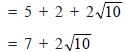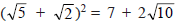(iv) (√5 – √2)(√5 + √2) = (√5)2 – (√2)2     [∵ (a + b)(a – b) = a2 – b2]
= 5 – 2 = 3
∴ (√5 – √2)(√5 + √2) = 3

Q.3. Recall, π is defined as the ratio of the circumference (say c) of a circle to its diameter (say d). That is, π = c/d. This seems to contradict the fact that π is irrational. How will you resolve this contradiction?
Ans.
When we measure the length of a line with a scale or with any other device, we only get an approximate rational value, i.e. c and d both are irrational.
c/d  is irrational and hence π is irrational. Thus, there is no contradiction in saying that π is irrational.

Q.4. Rationalise the denominators of the following:
(i) 1/7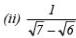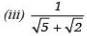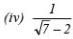Ans.
(i)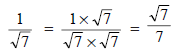(ii)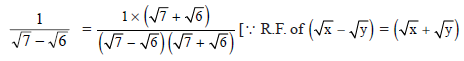Thus,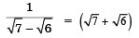(iii)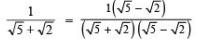[∵ RE of (√x + √y) is (√x - √y)]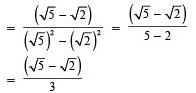Thus,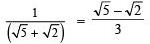(iv)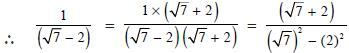[∵ R.F. of (√7 - 2) is (√7 + 2)]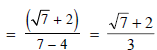Thus,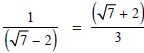Offer running on EduRev: Apply code STAYHOME200 to get INR 200 off on our premium plan EduRev Infinity!

,

,

,

,

,

,

,

,

,

,

,

,

,

,

,

,

,

,

,

,

,

;